1. Worksheets>
2. Math>
3. Number Sense>
4. Ordinal Numbers

Ordinal Numbers - Worksheets and Charts

Ordinal numbers worksheets have exclusive and numerous exercises and activities for children. Standard problems to write the ordinal numbers, interesting picture puzzles, exercises to differentiate ordinal and cardinal numbers, cut-paste activities and coloring activities are available in these worksheets. Colorful themed charts which carry ordinal numbers from 1 to 100 are displayed here.

Ordinal Number Charts

Ordinal number: Beginners charts

Interesting themed ordinal charts have the ordinals written in words and represented in numbers. Chart 3 is displayed with both ordinal and cardinal numbers.Ordinal number steps - 1st through 10th

Cardinal and Ordinal numbers - 1st through 20th

First through Fiftieth

First through Hundredth

Ordinal Number Puzzles

Picture puzzles: 1 to 5

Colorful and black-and-white picture strips are here in this puzzle. Cut them out, arrange them and paste the strips in the next page according to the ordinal numbers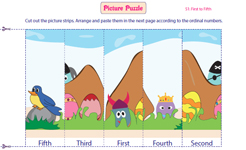In words (C):

In numerals (C):

In words (B/W):

In numerals (B/W):

Picture puzzles: 1 to 10

Try out these interesting and challenging puzzles. A picture is divided into ten parts. With the help of the given ordinal numbers arrange the picture strips and paste them in order.In words (C):

In numerals (C):

In words (B/W):

In numerals (B/W):

Ordinal Number Activities

Cut-paste activity

There are 10 students in a row. The first and the last student are already shown. The positions of the other six students are defined. Cut out the picture strips from the bottom and paste them according to the given instructions.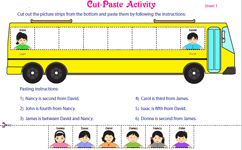Coloring objects

Five set of pictures are displayed in each worksheet. Each worksheet has an interesting theme for children. Color the picture which indicates the given ordinal number.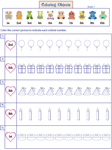Numerical Form:

Word Form:

Coloring activity

Exciting coloring activity with distinctive themes is here for your children. Follow the given instructions given and color accordingly.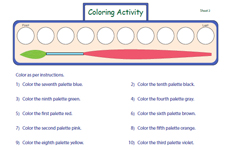Landscape:

Portrait:

Ordinal Number Worksheets

Cardinal or Ordinal

Each worksheet has seven statements given. Read each statement and recognize whether the number used in each statement is a cardinal or ordinal number.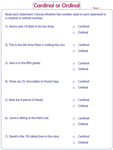Writing ordinal numbers

Six themed worksheets have numerous objects displayed in groups. One among them is colored. Write the ordinal number of the colored object.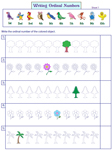Numerical Form:

Word Form:

Circle the objects: left / right

Each worksheet has six sets of objects shown. Circle the correct object according to the instructions given in each question.Identifying the position

Ten friends live on different floors of an apartment. Answer the questions in these themed worksheets to know on which floor they actually live on.Representing the position

Fill in the blanks with ordinal numbers to represent the position of common people who are standing in a bus stop, amusement park and for a photograph.Word form

Twenty standard problems are given here for practice. Part A has ordinal numbers written in words. Write the numerals for it. Part B has ordinal numbers to be written in words.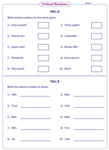Obtain the entire collection of worksheets on ordinal numbers in a jiffy!

Related Worksheets